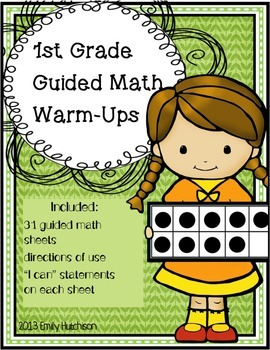# Daily Guided Math Warm-Ups: First Grade Edition1st
Subjects
Standards
Resource Type
Formats Included
• PDF
Pages
44 pages

### Description

Do your students need a quick warm-up before starting their guided math group math lesson each day? This packet contains templates for 1st grade Common Core math skills. They are meant to be teacher directed or collaborative sheets and can be used with a small group, at a center, or with a classroom of students. Each page can be put into a page protector, dry erase sheet, or laminated; therefore, they can be used over and over again. The teacher provides the problem and the student(s) fill in the rest or the students tell the teacher how to fill it in. This allows the teacher to differentiate the problems based on the need of the student. It also provides a quick review of previously learned skills.

The following skills are covered in the pack:
Telling Time
Geometry
10 more/less
1 more/less
Multiples of 10
Place Value
Missing Part
Story Problem
Fact Families
T/F Equations
Data/Graphs

There is an "I can" statement at the top of each template and the standard number is listed at the bottom. Each template includes a "suggested use" page for the teacher to reference.
Please download the preview to determine whether this would benefit your class of students!

emhutchison@gmail.com

Emily Hutchison

You may also find these products to be helpful:
Second Grade Guided Math SheetsFraction Introduction and SortsFREEBIE: Why Do We Need MathPlace Value SCOOT
Total Pages
44 pages
N/A
Teaching Duration
N/A
Report this resource to TpT
Reported resources will be reviewed by our team. Report this resource to let us know if this resource violates TpT’s content guidelines.

### Standards

to see state-specific standards (only available in the US).
Use addition and subtraction within 20 to solve word problems involving situations of adding to, taking from, putting together, taking apart, and comparing, with unknowns in all positions, e.g., by using objects, drawings, and equations with a symbol for the unknown number to represent the problem.
Solve word problems that call for addition of three whole numbers whose sum is less than or equal to 20, e.g., by using objects, drawings, and equations with a symbol for the unknown number to represent the problem.
Apply properties of operations as strategies to add and subtract. If 8 + 3 = 11 is known, then 3 + 8 = 11 is also known. (Commutative property of addition.) To add 2 + 6 + 4, the second two numbers can be added to make a ten, so 2 + 6 + 4 = 2 + 10 = 12. (Associative property of addition.)
Understand the meaning of the equal sign, and determine if equations involving addition and subtraction are true or false. For example, which of the following equations are true and which are false? 6 = 6, 7 = 8 - 1, 5 + 2 = 2 + 5, 4 + 1 = 5 + 2.
Determine the unknown whole number in an addition or subtraction equation relating three whole numbers. For example, determine the unknown number that makes the equation true in each of the equations 8 + ? = 11, 5 = ▯ - 3, 6 + 6 = ▯.

### Questions & Answers

Teachers Pay Teachers is an online marketplace where teachers buy and sell original educational materials.